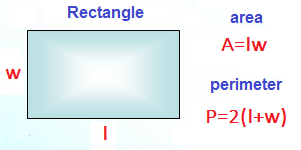## 9/13/2015

### How to find the area of a rectangle?

Here's a problem about the area of a rectangle of a textbook on algebra in 7 class (Russian school program):

If the width of the rectangle by 2 dm enlarge and reduce a length of 0.5 m, then we obtain a square, an area 50 dm² less than the area of the rectangle. Find the area of a rectangle.

Interestingly, in 7 class school studying systems of linear equations with two unknowns? Judging by the fact that the task of the textbook on algebra, so it is necessary to solve this problem. Stupidly make up the system of equations, bluntly we decide - anguish. If I'm here just write a solution, and you simply write off, the smarter you from it will not. I propose first to solve this problem, and the system of equations with the decision at the end we write.

What is square? It's a rectangle whose sides are equal. What is rectangle? It is a square, whose side are different. You are their teachers of mathematics do not govoriteovetuyu - for them it sounds like a desecration. I like "definitely" use constantly. After all, the mathematical properties of geometric objects they pass very accurately.

To solve the problem, we denote the side of the rectangle: a - the length, b - the width. Now we start again to read the statement of the problem.

"If the width of the rectangle to increase by 2 dm ...". In the language of mathematics it can be written as: b + 2.

"... And to reduce the length of 0.5 m ..." Here please pay attention - only completely illiterate people in a problem using different units of length. For example, meters and decimeters. We are educated people, as opposed to the author of the textbook, and translate everything in decimeters. Why decimeters? Because our area is measured in square decimetres. How many decimeters in one meter? That's right, ten. A 0.5 meter - decimeter is how many? Yes, 0.5 * 10 = 5 decimeters. Now we can take our phrase in the language of mathematics: a-5.

a-5=b+2

What does this leave us? Juggle this expression, we can express the length of one side through the length of the other side. In the future, it is useful to us. Personally, I do not like the "minus" sign. Now we get rid of him.

a-5=b+2
a=b+2+5
a=b+7

Something we digress from the conditions of the problem. Turn rewind and read the phrase: "... then we get a square with an area of 50 dm² is less than the area of a rectangle".How to find the area of a rectangle?

a*b-(a-5)*(b+2)=50
a*b-(a*b-5b+2a-10)=50
a*b-a*b+5b-2a+10=50
5b-2a+10=50
5b-2a=50-10
5b-2a=40

What's next? Now we can party instead of the original a substitute the result of juggling a=b+7.

5b-2a=40
5b-2(b+7)=40
5b-2b-14=40
3b=40+14
3b=54
b=18

The width of the rectangle we already know - 18 decimeters. We are looking for long.

a=b+7
а=18+7
а=25

Now we can easily determine the area of a rectangle 25 * 18 = 450 dm². The notebook can write all this as a system of two equations with two unknowns. I will give just two equations, choose any.How to find the area of a rectangle Decision.

On the left side, we expressed a square area over the length of the rectangle on the right side - in width. In the course of solving the problem, we have considered a third option - the area of the square is represented as the product of the length by the width. All three options provide the same result. Here on the use of mathematics equations to solve problems.